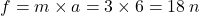## If the acceleration is 6m/s/s and the mass is 3kg, calculate the force? Include the UNIT

Question

If the acceleration is 6m/s/s and the mass is 3kg, calculate the force? Include the UNIT

in progress 0
2 months 2021-07-23T12:15:03+00:00 1 Answers 1 views 0